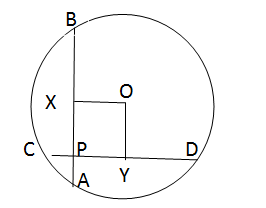# CAT Quantitative Aptitude Questions | Geometry Questions for CAT - Triangles

###### CAT Questions | CAT Geometry Questions | Circles

The question is from the topic circles. In this problem details about two perpendicular chords are given and we have to find out the radius of the circle. CAT Geometry questions are heavily tested in CAT exam. Make sure you master Geometry problems. Knowing chord intersection theorem helps.

Question 19: Two mutually perpendicular chords AB and CD intersect at P. AP = 4, PB = 6, CP = 3. Find radius of the circle.

1. 31.25(1/2)
2. 37.5(1/2)
3. 26(1/2)
4. 52(1/2)

## Best CAT Coaching in Chennai

#### CAT Coaching in Chennai - CAT 2022Limited Seats Available - Register Now!

##### Method of solving this CAT Question from Circles: Figure out the diagram and then the question becomes very easy.When 2 chords AB and CD intersect at P then AP * PB = CP * PD
Hence 4 * 6 = 3 * PD
Thus, PD = 8
Now AB = AP + PB = 10
And CD = CP + PD
Thus, CD = 11
Consider the circle with center O.
Drop a perpendicular from O to chord AB and CD.
This will bisect the chords at X and Y i.e AX=XB and CY = YD.
Here AX = AP + PX i.e 5 = 4 + PX
PX = 1
Similarly, since CD = 11, PY+CP+YD = 11,
=> PY = 11-3-5.5 = 2.5.
PY = OX and PX = OY.
So, PXOY will from a rectangle as seen in the figure.
Now consider the triangle BOX, it is a right triangle where OB is the radius.
XB = 5, OX = 2.5
Then OB = ( OX2 + XB2 )1/2 OB = 31.251/2 Thus radius = 31.251/2 The radius can also be found out using the triangle YOD.

The question is "Find radius of the circle."

##### Hence, the answer is 31.251/2.

Choice A is the correct answer.

###### CAT Coaching in ChennaiCAT 2023

Classroom Batches Starting Now! @Gopalapuram

###### Best CAT Coaching in Chennai Introductory offer of 5000/-

Attend a Demo Class

## CAT Preparation Online | CAT Geometry questions Videos On YouTube

#### Other useful sources for Geometry Question | Geometry Triangles Circles Quadrilaterals Sample Questions

##### Where is 2IIM located?

2IIM Online CAT Coaching
A Fermat Education Initiative,
58/16, Indira Gandhi Street,
Kaveri Rangan Nagar, Saligramam, Chennai 600 093

##### How to reach 2IIM?

Phone: (91) 44 4505 8484
Mobile: (91) 99626 48484
WhatsApp: WhatsApp Now
Email: prep@2iim.com